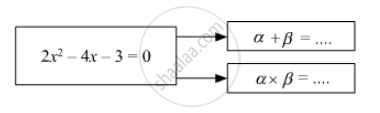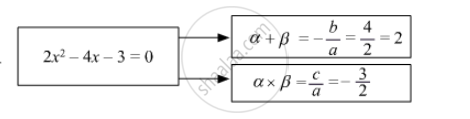# Fill in the Gaps and Complete.If α, β Are Roots of Quadratic Equation, - Algebra

Fill in the gaps and complete.

If α, β are roots of quadratic equation,#### SolutionSum of roots = $\frac{- b}{a} = \frac{- \left( - 4 \right)}{2} = 2$

Product of roots = $\frac{c}{a} = \frac{- 3}{2}$

Concept: Solutions of Quadratic Equations by Completing the Square
Is there an error in this question or solution?

#### APPEARS IN

Balbharati Mathematics 1 Algebra 10th Standard SSC Maharashtra State Board